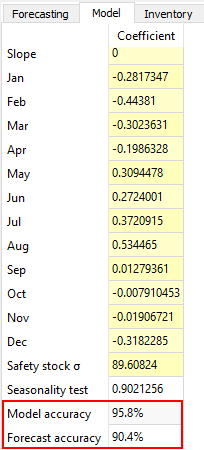#GMDH Streamline Docs

### Site Tools

evaluating-forecast-accuracy

# 5.10. Evaluating the Forecasts

It is always expected that actual values will deviate from the forecast. Measuring this difference as a KPI and assessing it for the safety stock calculation is an important part of demand forecasting process.

There are many error measures used to evaluate the forecast. Among them:

• Mean Squared Error (MSE);
• Root Mean Squared Error (RMSE);
• Mean Absolute Error (MAE);
• Mean Absolute Percentage Error (MAPE).

Streamline evaluates forecast based on MAPE. It is obvious that in order to evaluate the forecast the actual data for the forecasted period must be imported.

Consider an example. Assume that today is the end of Jul 2017 and we build forecasts 6 months ahead (see figure below).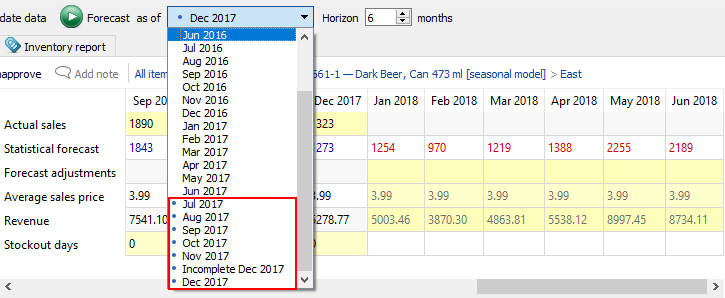After the forecasts have been generated, Streamline automatically assigns them to the currently selected As of period. At the end of the next month – Aug 2017 – we generate the forecasts again. And so on. Let’s keep doing this until the end of Dec 2017. In the result, we have forecasts for 6 periods starting from Jul 2017 to Dec 2017.

Now, when we have actual data up to Dec 2017, let’s evaluate the forecasts generated as of Jul 2017. To do this, select this period in the As of control. Let’s look at the item Dark Beer in the East location (see figure below).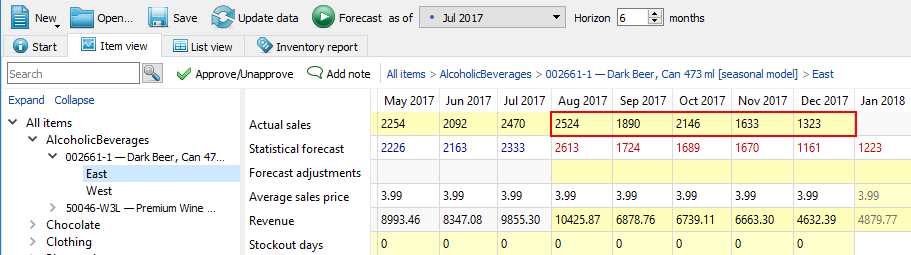As you see, Streamline automatically loads and shows all available actual data regardless of the period selected in the As of control (see figure above) and evaluates the forecasts against this data.

## Viewing Forecast Quality

The results of the forecast evaluation are represented by the Model MAPE and Forecast MAPE in the Model tab of the Panel (see figure below).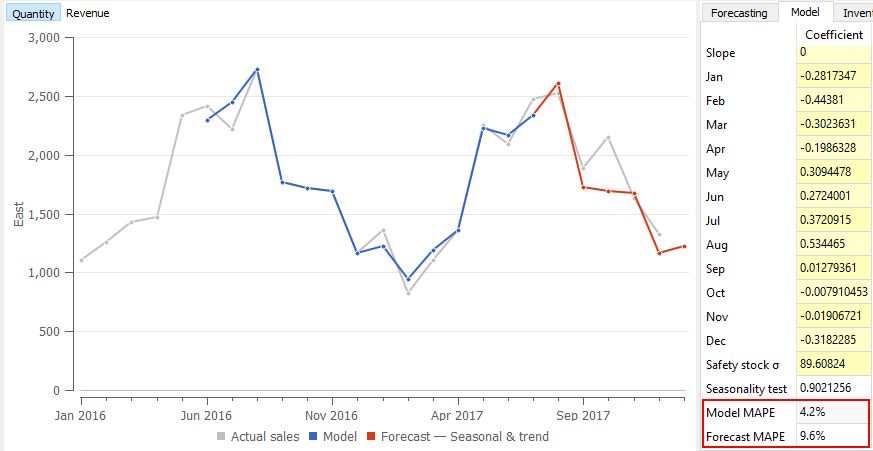• Model MAPE is MAPE calculated based on the model response for the data that was used to build the model. This response is indicated with the blue dots in the Plot (see figure above).
• Forecast MAPE is MAPE of the forecast. It is calculated if there is actual data to evaluate the forecast against.

The Forecast MAPE shown in the figure above is the evaluation of five periods (Aug 2017 - Dec 2017) for which actual data exists.

To see the forecasts evaluation for all of the items in one report, go to the Reports tab and select the Forecast error report (see figure below).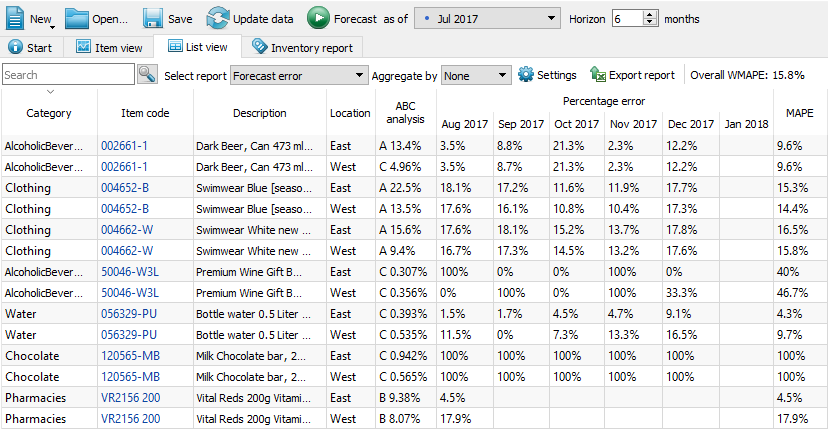The Absolute percentage error section of the report represents APE calculated for each item, location, and period. As we do not have actual data for Jan 2018, the corresponding column is empty in the report.

The table can have gaps or even empty rows. A gap occurs when there were no actual sales in the period. Streamline can’t calculate APE in this case. An empty row arises if either the item is inactive or an intermittent model is used.

The last column of the report represents MAPE calculated across all of the periods for each planning item.

The Overall MAPE, shown in the toolbar, is MAPE weighted across all the items in the project. If the products’ prices are given this is a price-weighted MAPE measure that represents overall revenue percentage error. Otherwise, it is calculated based on the volume of sales. You can read more about WMAPE on Wikipedia.

You can also switch the measure of the forecast quality between error and accuracy (which is 100% - error ). To do this, we click the Settings button found on the toolbar and select the Accuracy option for the Measure of forecast quality (see figure below).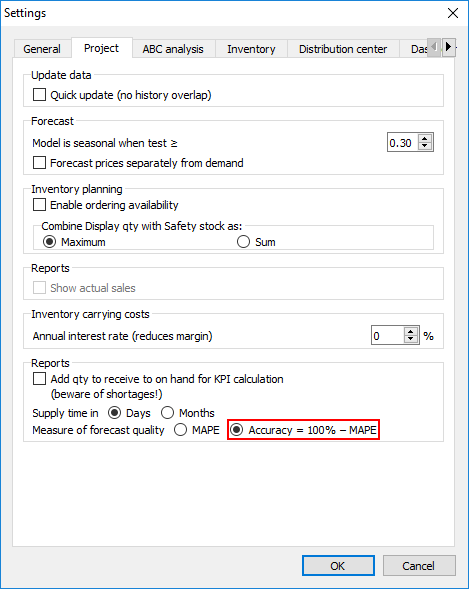Now, the report shows the percentage accuracy of the forecasts for each period in the Percentage accuracy section and the average accuracy across the available evaluations in the last column (see figure below).

The Overall accuracy of the toolbar equals to 100% - Overall MAPE.

2018/04/02 14:55

## Forecast Quality Measures

Streamline is able to evaluate forecast quality using two measures:

• Error (MAPE) or
• Accuracy (100% - Error).

The default measure is Error. You can switch between the measures by going to the menu File > Settings > Project tab > KPIs group and changing the Measure of accuracy parameter (see figure below).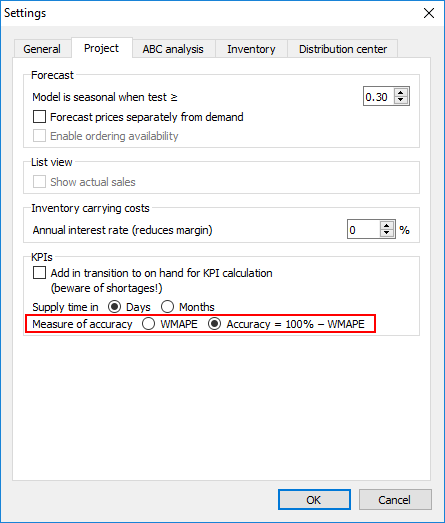The figure below shows the Forecast accuracy report when the measure is set to Accuracy in the Settings.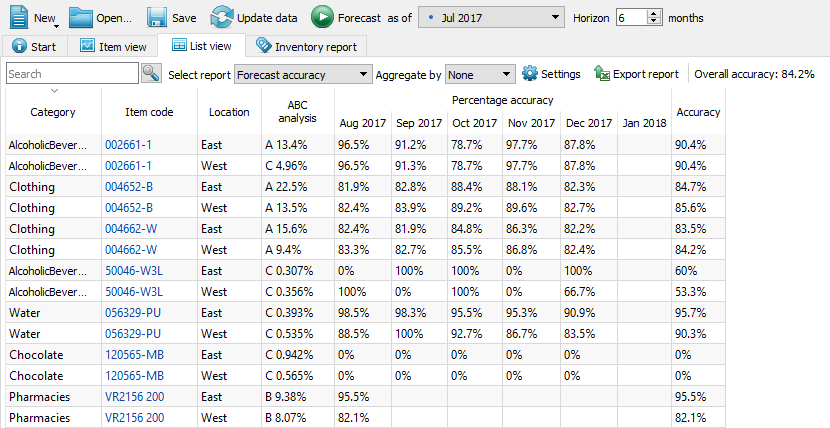This option also affects the evaluation shown in the Model tab of the Demand forecasting (see figure below).